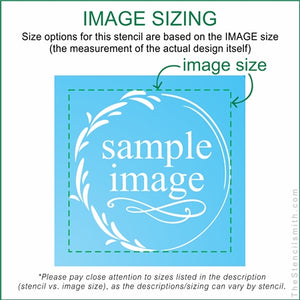# 546 - GAME BOARD (primitive edging)## The Stencilsmith

\$10.95

GAME BOARD SIZES:
*This is sized by actual image size. IMAGE size is the design itself (shown in black), STENCIL size is the rectangle around it

6" - STENCIL measures 7 1/2" x 7 1/2" - Actual GRID measures 6" x 6" (squares are .7")

7" - STENCIL measures 8 1/2" x 8 1/2" - Actual GRID measures 7" x 7" (squares are .81")

8" - STENCIL measures 9 1/2" x 9 1/2" - Actual GRID measures 8" x 8" (squares are .93")

9" - STENCIL measures 10 1/2" x 10 1/2" - Actual GRID measures 9" x 9" (squares are 1.05")

10" - STENCIL measures 11 1/2" x 11 1/2" - Actual GRID measures 10" x 10" (squares are 1.15")

11" - STENCIL measures 12 1/2" x 12 1/2" - Actual GRID measures 11" x 11" (squares are 1.28")

12" - STENCIL measures 13 1/2" x 13 1/2" - Actual GRID measures 12" x 12" (squares are 1.39")

13" - STENCIL measures 14 1/2" x 14 1/2" - Actual GRID measures 13" x 13" (squares are 1.5")

14" - STENCIL measures 15 3/4" x 15 3/4" - Actual GRID measures 14" x 14" (squares are 1.62")

15" - STENCIL measures 17" x 17" - Actual GRID measures 15" x 15" (squares are 1.74")

16" - STENCIL measures 18" x 18" - Actual GRID measures 16" x 16" (squares are 1.86")